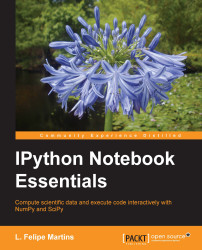•#### IPython Notebook Essentials#### Overview of this book

IPython Notebook EssentialsCreditswww.PacktPub.comPrefaceFree Chapter
A Tour of the IPython NotebookThe Notebook InterfaceGraphics with matplotlibHandling Data with pandasAdvanced Computing with SciPy, Numba, and NumbaProIPython Notebook Reference CardA Brief Review of PythonNumPy ArraysIndex## Computational and graphics tools

The objects of pandas have a rich set of built-in computational tools. To illustrate some of this functionality, we will use the random data stored in the `dframe` object defined in the previous section. If you discarded that object, here is how to construct it again:

```means = [0, 0, 1, 1, -1, -1, -2, -2]
sdevs = [1, 2, 1, 2,  1,  2,  1,  2]
random_data = {}
nrows = 30
for mean, sdev in zip(means, sdevs):
label = 'Mean={}, sd={}'.format(mean, sdev)
random_data[label] = normal(mean, sdev, nrows)
row_labels = ['Row {}'.format(i) for i in range(nrows)]
dframe = DataFrame (random_data, index=row_labels)
```

Let's explore some of this functionality of the built-in computational tools.

• To get a list of the methods available for the object, start typing the following command in a cell:

```dframe.
```
• Then, press the Tab key. The completion popup allows us to select a method by double clicking on it. For example, double click on `mean`. The cell text changes to the following...